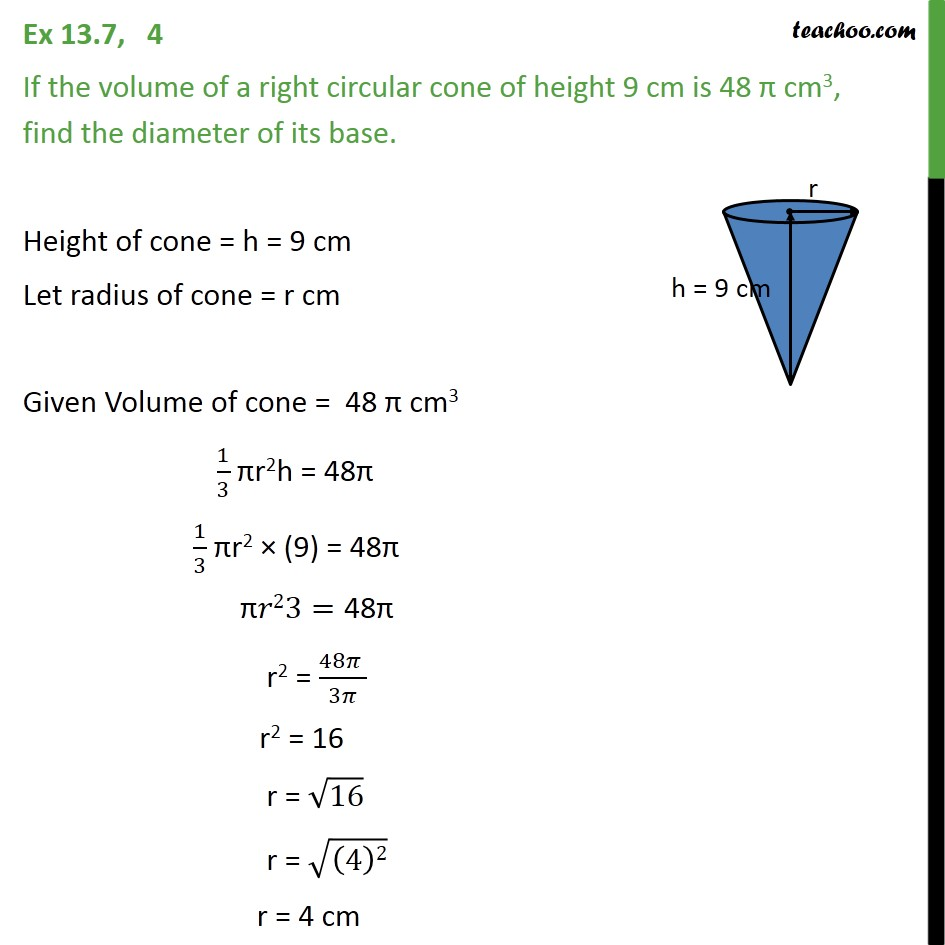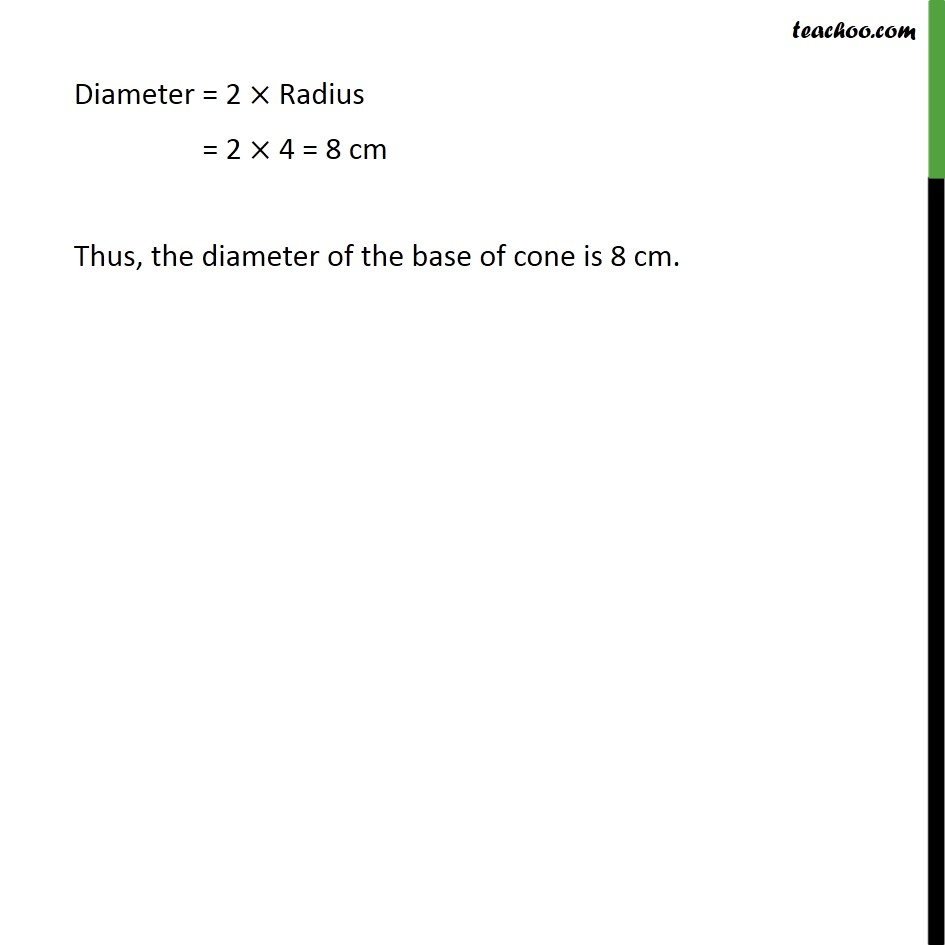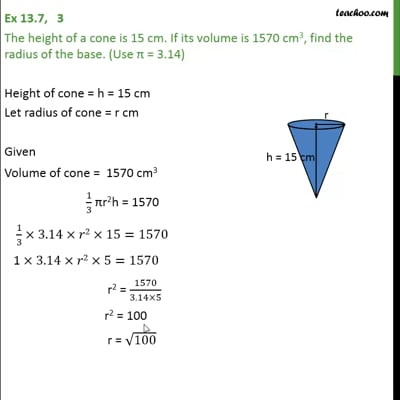Volume Of Cone

Chapter 13 Class 9 Surface Areas and Volumes
Concept wiseThis video is only available for Teachoo black users

Introducing your new favourite teacher - Teachoo Black, at only ₹83 per month

### Transcript

Ex 13.7, 4 If the volume of a right circular cone of height 9 cm is 48 cm3, find the diameter of its base. Height of cone = h = 9 cm Let radius of cone = r cm Given Volume of cone = 48 cm3 1/3 r2h = 48 1/3 r2 (9) = 48 " " 23="48 " r2 = (48 )/3 r2 = 16 r = 16 r = ((4)2) r = 4 cm Diameter = 2 Radius Thus, the diameter of the base of cone is 8 cm.